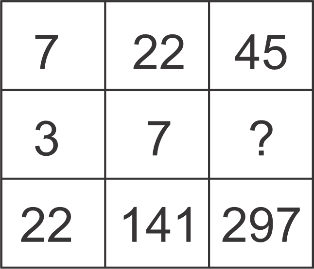Question of The Day20-08-2020

Study the pattern carefully and find which of the following option will replace the missing term?Correct Answer : d ) 12

Explanation :

Observing the pattern in the columns of the table we get

The general logic for each column is

(Term in Row 1)2 – (Term in Row 2)3 = (Term in Row 3)

Therefore, for column I

(7)2 – (3)3 = 49 – 27 = 22 (Third term)

For column II

(22)2 – (7)3 = 484 – 343 = 141 (Third term)

Now, for column III

(45)2 – (X)3 = 297

X3 = 2045 – 297

X3 = 1728

X = 12

Hence, (d) is the correct answer.

Such type of question is asked in various government exams like RRB JE, RRB NTPC, RRB GROUP D, UPSC CDS, UPSC NDA, UP SI etc.

Read Daily Current Affairs, Banking Awareness, Hindi Current Affairs, Word of the Day, and attempt free mock tests at PendulumEdu and boost your preparation for the actual exam.0